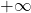# Functions

In the previous lesson, you learned about Cartesian coordinate system, graph of equations and linear equations. These are prerequisites to learn about function in this lesson.

A function is a relation between two sets – set A and set B, where each element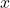of set A is related to exactly one element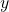of set B. Note the word “exactly” which is very important.

The elementsis called domain of function (or inputs) and the elementsis called the range of function( or outputs).

## Rules for Function

A function must follow certain rules, otherwise it is not a function.

1. Every element of set A must be assigned to an element of set B.
2. Each element of set A must match with an element in set B.
3. An element of set B can be output of two or more elements of set A.
4. An element of set A cannot be input of two or more elements of set A. (See rule 2).

### What are the different ways to represent functions ?

There are 3 ways to represent functions.

Numerically – you can represent a function as set of ordered pairs (x, y) where x is the domain of the function and y is the range of the function.

For example, you can represent above diagram as

{(1, A), (2, B), (x, y)}

Algebraically – you can represent a function using algebraic notations. A function is denoted as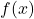.

For example, you are given an expression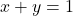.

The above expression can be changed to following form

y = 1 - x

or

f (x) = 1 - x

Graphically – you can represent a function using graph. A reveal lot of information about function such a increasing function, decreasing function, odd or even and other characteristics.

To represent a function using graph find the points required to plot the graph of the function. This is done using algebraic expression of the graph.

For example, let’s draw the graph of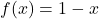.

The graph of the function using above points is given below.

## Piecewise-Defined Functions

A domain of a function is all real numbers. Sometimes a function is piecewise-defined. It means there are more than one function for different values of domain.

You need to specify two or more equations to represent a function with specific domain values.

For example,

f(x) = x2-1x<0x -1x≥0

Points to Remember

• If x value falls under the domain, then the function f(x) is defined for x and if x does not falls under the domain of f(x) then it is undefined for x.
• When the domain of the function is not mentioned then the implied domain is set of all real numbers.
• The range of the function must be a possible value after inserting x into the function f (x). The range falls between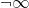to